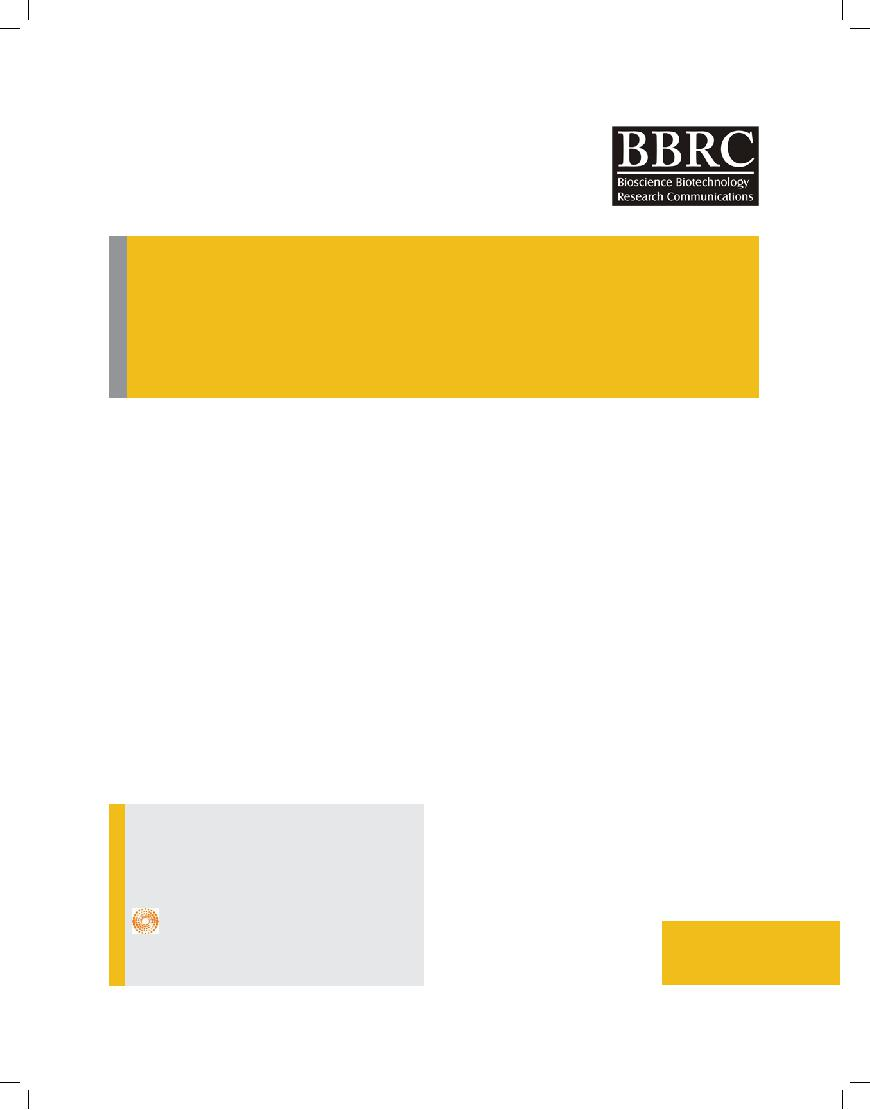Biotechnological

Communication

Biosci. Biotech. Res. Comm. 9(1): 46-54 (2016)

Determination of optimal combination of applied water and nitrogen for potato yield using response surface methodology (RSM)

Department of Irrigation and Drainage Engineering, Aburaihan Campus, University of Tehran, Tehran, Iran

ABSTRACT

Irrigation water has become less available in many regions due to global climate change and an increased competition for water by industry, domestic purposes, and the environment. To confront this challenge, there is an urgent need to develop water-saving irrigation strategies and techniques in order to maximize crop water use efficiency (WUE). In the other hand over-fertilization of mobile nutrients like nitrogen (N) may lead to leaching beyond root zone under plentiful irrigation, while it may decrease yield under limited irrigation. Thus, fertilizer N needs to be optimized in relation to water availability. In order to determine optimum levels of water and nitrogen of potato, a field experiment was carried out by central composite design (CCD) as Response surface methodology (RSM) as a most popular optimization method, in Dehgolan plain in Kurdistan province, west of Iran, spring season of 2014. The treatments were designed based on low and high levels of water supply (50% and 100% of crop water requirement) and applied nitrogen (250 and 350 kg N ha−1) as independ- ent variables. In this study, tuber yield (Y) and WUE were measured as response variables. Optimum levels of N fertilizer and irrigation depth were determined based on three scenarios: availability and nonavailability of water for irrigation. Furthermore, for environmental consideration, effects of minimum rate application of N on surface responses were evaluated also.. The results showed a marked interac- tion between level of irrigation and nitrogen on potato Y and WUE. The results also revealed that increasing irrigation and fertilizer led to an increase in tuber yield. WUE was decreased with increasing in water supply but N increasing had positive effect on WUE in a certain irrigation depth. Maximum Y was 69200 kg ha−1, while application of 287 mm irrigation and 350 kg N ha−1 was led to maximum WUE (159 kg ha−1 mm−1). Based on response surfaces of Y and WUE, the amounts of 552 mm and 349.5 kg N ha−1 was found to be the optimum levels of water and nitrogen for unlimited water conditions. WUE value in this situation was 130 kg ha−1 mm−1. For scarce water condition such as arid and semiarid regions, optimum values of irrigation water and N based on response surfaces were simulated 287 mm and 350 kg N ha−1, respectively. If the goal of optimization is maximizing Y and WUE simultaneously, then applied water and nitrogen was estimated about 461 mm and 350 kg N ha−1 and corresponding Y and WUE were achieved 62742 kg ha−1 and 143 kg ha−1 mm−1 respectively. For minimizing N application, optimum levels of resources were found 502 mm irrigation and 250 kg N ha-1. In this scenario 50242 kg ha−1 for Y and 105 kg ha−1 mm−1 for WUE were obtained. Comparison of results showed that WUE maximizing strategy resulted in 48% saving in water supply. Simultaneous maximizing of Y and WUE reduced applied irrigation water by 17% and consequently tuber yield reduction rate was 9%. Potato yield reduction in minimum use of N was 27% while irrigation water applied decreased by 9%.

KEY WORDS: NITROGEN, OPTIMIZATION, POTATO, RESPONSE SURFACE METHOD

ARTICLE INFORMATION:

*Corresponding Author: Jsoltani@ut.ac.ir Received 2nd February, 2016

Accepted after revision 28th March, 2016 BBRC Print ISSN: 0974-6455

Online ISSN: 2321-4007

Thomson Reuters ISI SCI Indexed Journal NAAS Journal Score : 3.48

© A Society of Science and Nature Publication, 2016. All rights46 reserved.

Online Contents Available at: http//www.bbrc.in/INTRODUCTION

Water availability is the most important factor limiting agricultural development in arid and semi- arid regions. Unbalanced distribution of water and nutrients, led sig- nificantly to decrease the crop yield Researches have shown that the efficient combination of soil moisture and fertilizer considered as the most important issue to improve economic and ecological output of crop pro- duction and to ensure sustainable production in dry land agriculture. According to FAO reports, potato (Solanum tuberosum L.) with 368 million tons production poten- tial from 3.19 million hectares under cultivation has an important role in the world agriculture. It is also one of the most important vegetable commodity in Iran, har- vested about 56.4 million ton of 153 thousand hectares under cultivation. Water and nitrogen are two key fac- tors in potato production (Yuan et al., 2015; Wanddell, 1999 Deng et al., 2006, Meyer & Marcum, 1999) where as some studies found strong interaction effects between these two factors on potato yield, (Hou et al., 2012, Alva, 2012; FAO, 2012, Ierna, 2013, Fu et al., 2016).

Based on Fu et al. (2016) the effect of water and ferti- lizer on plant growth is synergistic. On the one hand low efficiency of irrigation will limit the effect of fertilizer on plant growth, so that using too much water leads to a decrease in yield and nitrate leaching. On the other hand to apply excessive amounts of nitrogen or less of it will affect on water use efficiency and thus reduce the yield (Zhai & Li, 2014, Fu et al., 2016).

Shen et al. (2010) have demonstrated that a fixed amount of irrigation water and the optimum amount of fertilizer leads to maximum performance as well as in a fixed amount of fertilizer the optimal amount of irriga- tion water determines maximum performance, therefore optimal amount of water and fertilizer coupling is prob- ably the best way to improve water and nitrogen use efficiency. Of the objectives of agricultural development in arid and semi-arid areas are to achieve high yield and water use efficiency, hence the analysis and evaluation of the optimal amounts of water and fertilizer used to enhance the crop yield and water use efficiency has a great role on saving water in agriculture (Liu & Zhang, 2015; Liu et al., 2012 and Zhai & Li, 2015).

Recently response surface methodology (RSM) is presented as an effective way for solving optimization problems. Koocheki et al. (2014) used of the RSM to determine the optimum values of water, nitrogen and the crop density in canola cultivation. The results showed that increasing the amount of irrigation water and ferti- lizer leads to increased yield and nitrogen losses, while increasing crop density increased the yield and reduce nitrogen loss. In another study in order to optimize the input water, applied nitrogen and crop density of onion

using response surface methodology, results showed that applying 93.48 kg N ha-1 and 8930 m3 of irrigation water with 42.6 density of plants per m2 is the best combina- tion (Mansouri et al., 2014). Potatoes, wheat and alfalfa are the dominant plants cultured in Kurdistan province. More than 95% of the under cultivation areas of potato are in the Qorveh and Dehgolan plains. Hosseinpanahi et al., (2012) in their analysis studied several important crops in the Kurdistan province and prediction of its production in a recent program and found that potato cultivation will increase about 42.4%. therefore in order to preserve the needed water for the under potato cul- tivation areas, as well as to apply appropriate amount of nitrogen preventing available aquifer pollution, it is necessary to apply water and nitrogen so as to optimize both production and water use efficiency.

The aim of this paper was to study the effect of dif- ferent amounts of irrigation water and nitrogen fertilizer on yield and water use efficiency, determining optimum levels of irrigation and nitrogen based on three sce- narios: limited, unlimited water resources and applying least amount of nitrogen fertilizer as well as to predic- tion the response surface values corresponding to irri- gation water and nitrogen values in potato production using response surface methodology based on central composite design (CCD) in Kurdistan province.

MATERIAL AND METHODS

RESPONSE SURFACE METHODOLOGY (RSM)

Because of the wide range of treatments combination (combination of different levels of water and nitrogen) using multi- factor experiments to assess their impact as well as selecting the best combination treatment is costly, complex, time consuming and associated with experimental errors . Therefore RSM has been suggested to determine the effects of individual factors and the effects of their interaction (Koocheki et al., 2014).

Using response surface methodology, the interactions between factors likely influencing on the response by a limited number of experiments can be assessed (Wang et al., 2014). RSM is a statistical method based on mul- tivariate non-linear model that can be used to optimize the resources. In order to evaluate exactly the response that obtained of the fitness of observational data, as well as determining the optimal amounts of independent variables, this method is applicable to several different experimental designs (Kalavathy et al., 2009; Mansouri, 2014).

RSM assesses the responses by simultaneous chang- ing several factors and using a limited number of exper- iments. So this method is a powerful tool for statisti-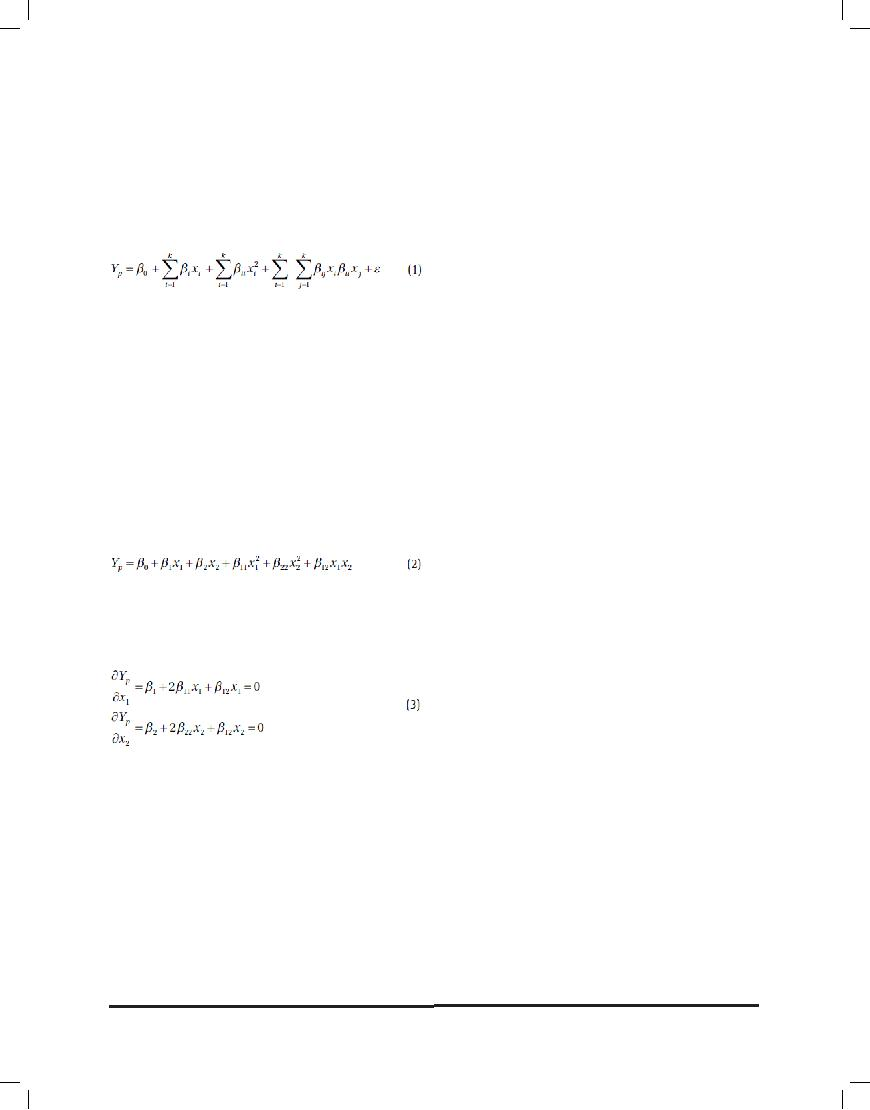cal simulation and optimization available variables that while reducing the number of treatments, on the one hand reduce experimental errors and on the other hand save significantly on operating costs by spending less time. In this study, observational response surface data was fitted based on CCD tests using the following quad- ratic polynomial equation:

Where Yp represent predicted responses, β0, βi, βii, βij represent constant term, linear, squared and interactions expression coefficients respectively. and are Independent variables, k is the number of variables that in this study k = 2 and ε is the error of estimation. Fitted equation is used to achieve optimal levels of consumption as well as the corresponding values of the response surfaces. Based on mathematical analysis, the optimal point of response surface that is also called the stationary point (maximum or minimum) of a quadratic equation, one that has no function, where the first derivative is zero. In this study, two independent variables (water and nitrogen fertilizer) and also two dependent variables (water use efficiency and yield) were studied. The response surface equation would be as follow:

As noted, with derivation to this procedure the optimum points of the function will be obtained. The following equations show the derivative with respect independent variables:

Where x1 is irrigation water depth (mm), and x2 is nitrogen (kg ha-1). By solving simultaneous linear equations 3 x1 and x2 optimal values are achieved.

RSM has several advantages in comparison to clas- sic experiments or optimization procedures. First, in this method researchers using a few tests obtain large amounts of information. In contrast, classical meth- ods are time consuming and require a large number of experiments to explain the behavior of a system. Another advantage of RSM is that in this method it is possible to observe the interactions of independent parameters on the responses. On the other hand, the most important weakness of the RSM is being fitted to a quadratic poly- nomial. Because you cannot say all systems are nonlin-

ear behavior are accommodated by quadratic polyno- mials. In such cases, especially in asymmetric systems, can used quadratic polynomial equation with data con- version to other forms (logarithmic conversion, sinusoi- dal conversion, etc.). Another solution to overcome this problem is to reduce the number of input parameters (independent variables). In this case, it should be noted that reducing the number of independent parameters decreases the probability of determining the exact sta- tionary point (optimal point) (Denis & Boyac, 2007). In this study, to design the experiment as well as to evalu- ate and optimize the resources, Design Expert software (version 7.0.0) was used.

LAYOUT OF EXPERIMENTAL DESIGN BASED ON CCD

Central composite design is a perfect replacement for the factorial design which was developed originally by Box and Wilson (1951), and then was improved by Box and Hunter (1957). The CCD provides data equivalent to three- level factorial, but by reducing the number of experiments (less than full factorial experimental) and also arranges experiments with different combinations of the independent variables (Koocheki et al., 2014). Table 1 shows maximum, minimum and average values limits of independent variables and their coded values. As could be seen CCD matrix includes 13 runs.

The field experiment was conducted in the spring of 2014 in a farm located in Dehgolan Plain, Kurdistan province, geographically located at (35°12’N, 47°37’E) and 1895 meters above the sea level. The amount of Acidity and electrical conductivity of the irrigation water were 7.49 and 0.57 ds m-1 respectively as well as farm soil texture was silt clay with the bulk density equal to 1.37(gr cm-3). Some physical and chemical properties of the soil are shown in Table 2.

The minimum and maximum irrigation water depth was selected based on low and high levels of water supply (50% and 100% of crop water requirement) and applied nitrogen (250 and 350 kg N ha−1) as independ- ent variables. +1,-1 and 0 are dimensionless coded val- ues of the independent variables. Amounts of water and fertilizer for experimental treatments based on the CCD method are shown in Table 1.

Water requirement calculated using climate data and Cropwat 8.0 software. Half of nitrogen fertilizer added to the soil before planting by hand, and the remainder in the tuber formation stage injected in pipes of drip irrigation system (fertigation). Plots were established in 15 meters long and 5 meters wide with four rows spaced 75 cm. Plots were separated 2 m from each other. Irriga- tion treatments were performed using subsurface drip irrigation (SDI). For this purpose, drip irrigation tapes in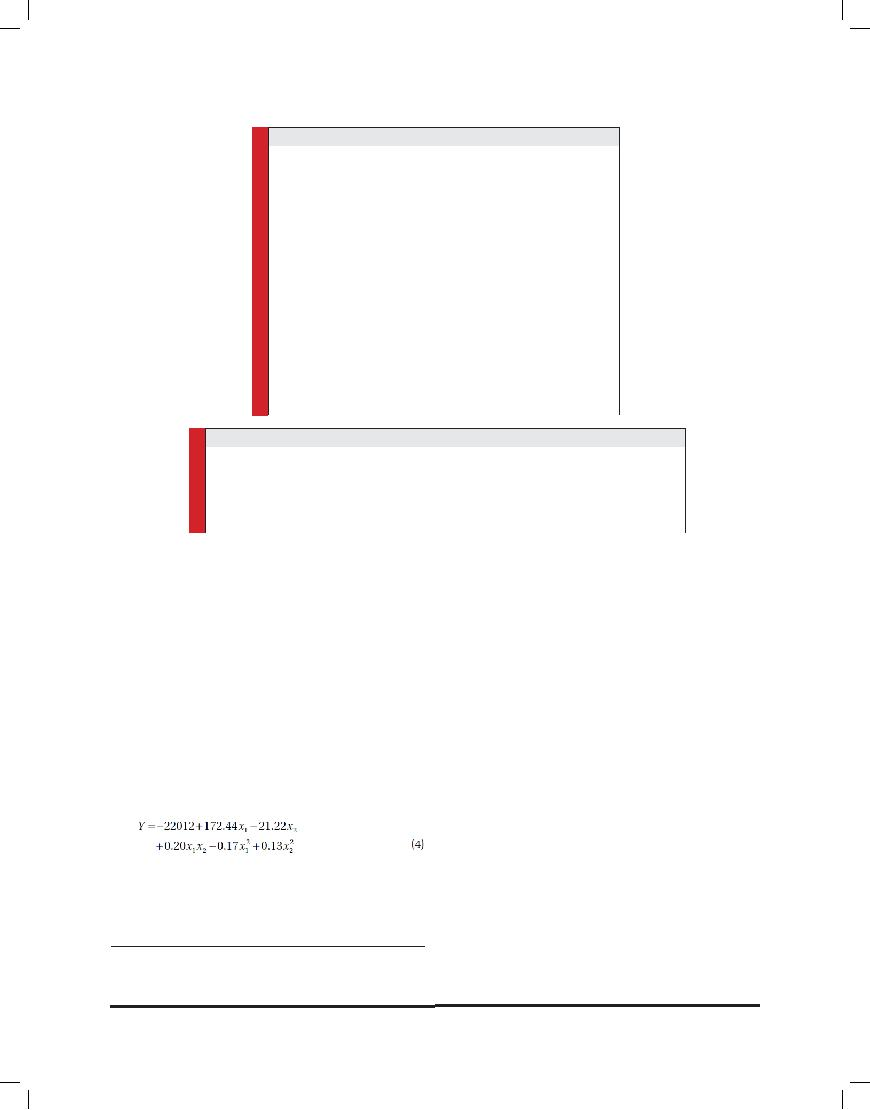Table 1: Actual and coded levels of independent variables

Table 2: Physical and chemical properties of soil

15 meters length and 16 mm inner diameter, were posi- tioned with drippers at 20 cm intervals at a depth of 10 cm from the row hill.

For measuring and controlling the amount of irri- gation water, volumetric flow meters and polyethylene control valves were used. Two days fixed irrigation inter- val was accomplished. All agricultural operations such as weeding and spraying were performed equally in all plots. The primary irrigation for growing potatoes was arranged equal to 20 mm in all treatments. At the end of the growing season tuber yield values were measured. In order to eliminate the marginal effects, only the middles of 10 meters between two rows of each treatment were harvested. The following equation was used to calculate the amount of water use efficiency (Howell et al., 1990):

Where Y is the yield ((kg ha-1)) and ET seasonal crop evapotranspiration (mm).

criteria were used. Determination coefficient is a meas- ure of the strength of the linear relationship between two variables, which at best should be equal to 1. Lack of fit test calculations obtain by comparing the sum of the remaining squares and the sum of the squares (F-test) which non significance of this parameter indi- cates the appropriateness of the model (Mansouri et al., 2014; Koocheki et al., 2014).

Table 3 represents the details of the analysis of the quadratic polynomial model variance fitted for yield response and water use efficiency response variables. Using ANOVA table a full quadratic polynomial equa- tion was obtained and all its components other than the

square term of nitrogen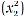were significant (P <0.01) based on the F test for the Y and WUE.

It was also observed that the lack of fit criterion for both yield polynomial model and water use efficiency was not significant; suggest that the model explained experimental data satisfactory. The values of determina- tion coefficients also show that the results of the fitted model and measured values had a strong positive lin- ear correlation (Table 3).

RESULTS AND DISCUSSION

For assessing the model and judgment about the fitted model, determination coefficient (R2) and Lack-of-fit

YIELD OF POTATO

Maximum Potato tuber yield was achieved 69,200 kg per hectare in full water requirement treatment By applica-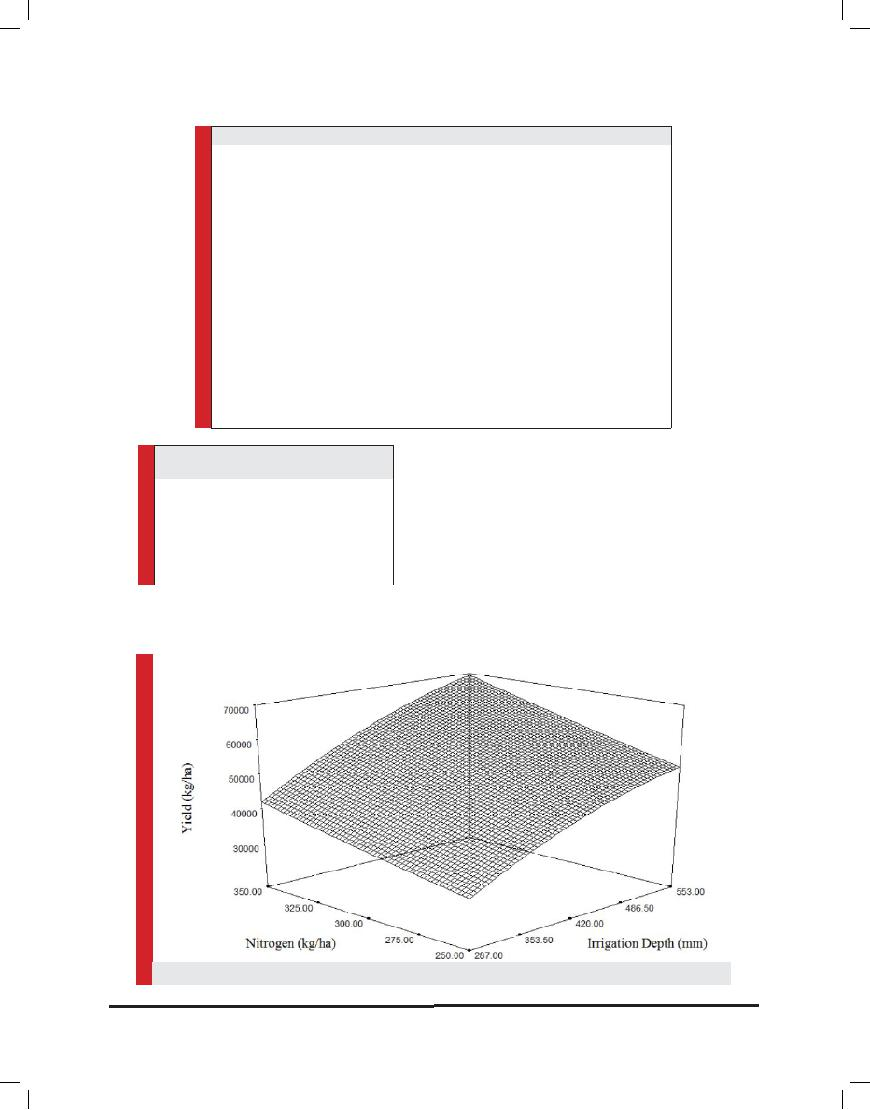Table 3: ANOVA table for fitted models

x2: Applied nitrogen fertilizer; “s”: significant variables and “ns”: Non significant variables. x1: Applied irrigation depth

Table 4: Maximum and minimum values of Yield and water use efficiency (WUE)

tion of 553 mm Irrigation water and 350 Kg N ha-1. Also according to Fathi and Soltani (2012) maximum perfor- mance was obtained in full irrigation. While the minimum yield was obtained using of 287 mm of input water and 250 Kg N ha-1 fertilizer at a rate of 30850 Kg ha-1 (Table 4).

The results showed that reducing the amount of irri- gation water and fertilizer lead to a decline in potato yield (Figure 1). The decline in yield due to deficit irriga- tion was expected which according to the investigators (Badr et al., 2012) was due to plant reduced evapotran- spiration. On the other hand, according to the findings of the researchers, since using nitrogen leads to spread the

FIGURE 1: The yield response (kg/ha) to applied irrigation depth (mm) and nitrogen use (kg/ha).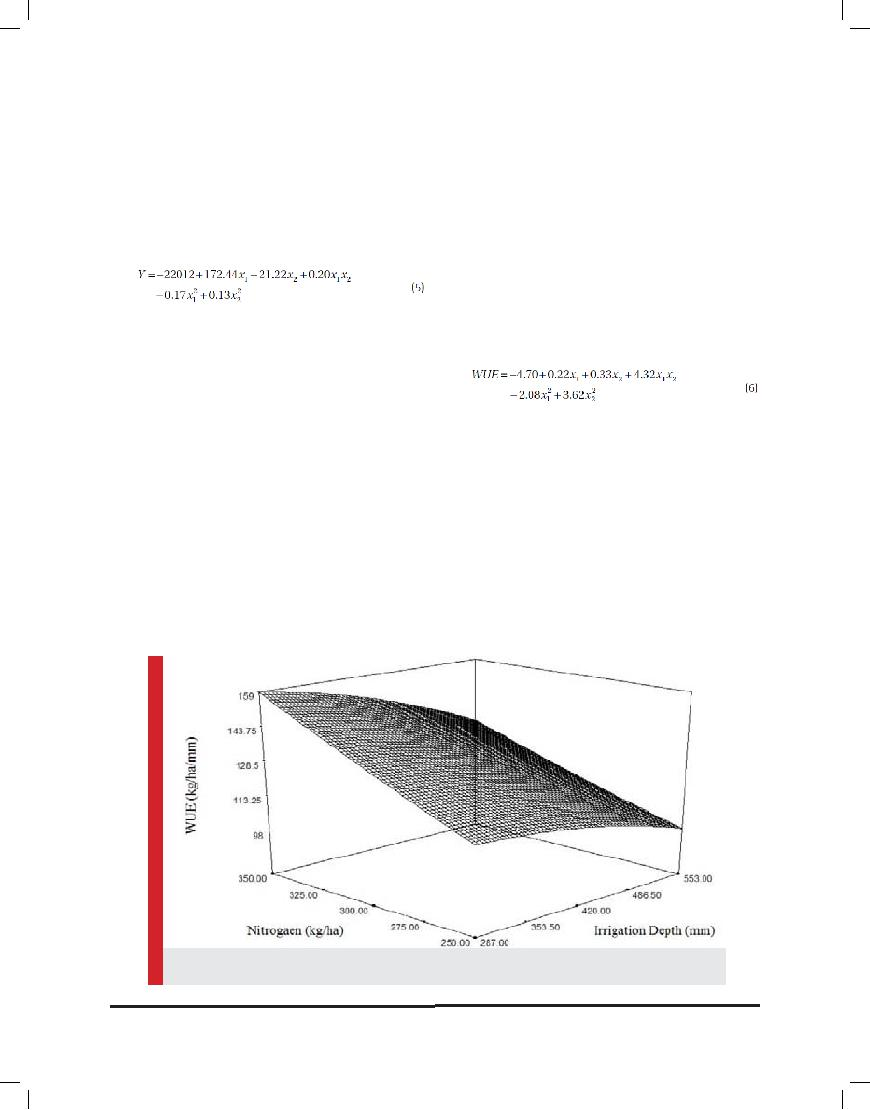roots and improve plant growth (Mansouri et al., 2014; Koocheki et al., 2014) therefore reducing N amounts decrease the performance too. According to Figure 1 it is clear that reducing of input resources, lead to yield loss, also the tuber yield reduction slope is more when reduc- ing water than reducing nitrogen.

Quadratic polynomial equation fitted to the yield actual values was determined as:

Where Y is yield response surfaces, x1 irrigation depth (mm) and x2 nitrogen fertilizer (kg / ha).

According to the coefficients in the equation of the yield response surfaces it is clear that the impact of water shortages on yield loss is greater than reduction of nitrogen. So we can say that in deficit irrigation con- dition, increases in nitrogen is ineffective. So this find- ing is in accordance to Badr et al.and colleagues (Badr et al., 2012). Researchers interpreted the reason in terms of water stress. deficit irrigation condition hampered the transfer of nitrogen to the root and lead to a sharp decline in its growth. Therefore in terms of water short- age or deficit (irrigation), nitrogen is not a limiting fac- tor for potato production, the determining factor is the amount of water (Badr et al., 2012; Darwish et al., 2003).

gen fertilizer (Table 4). Table 4 show minimum and maxi- mum values of water use efficiency. The results showed that in deficit irrigation treatments, water was used in the most effective manner as could be seen in the results of other researchers (Pandey et al., 2003). So that applying the minimum amount of water, lead to the maximum water use efficiency. The maximum water use efficiency obtained with the use of 287 mm of irrigation water and 350 kg nitrogen per hectare. Considering the changing trend of water use efficiency in nitrogen treatments it can be seen that a reduction in applied irrigation depth increases water use efficiency. This is clearly visible in Figure 2.

The equation of water use efficiency response to the used resources is as follows:

Where WUE are water use efficiency response levels, x1 is irrigation water depth (mm) and x2 nitrogen fertilizer (kg N ha-1). Results of the water use efficiency values showed that in a fixed amount of water applied increas- ing nitrogen rate increased water use efficiency. This is due to the positive effect of nitrogen on potato yield, the finding that is in compliance to the results of other stud- ies (Badr et al., 2012; Kashyap & Panda, 2003).

WATER USE EFFICIENCY

Water use efficiency in potato, which is obtained of the tuber yield compared to seasonal crop evapotranspiration (ETc), have wide variations in levels of irrigation and nitro-

OPTIMIZATION OF INPUT WATER AND NITROGEN FERTILIZER

In this study to determine the optimal amount of input water and nitrogen, as well as to find the corresponding

FIGURE 2: The Water use efficiency response (kg ha-1 mm-1) to applied irrigation depth (mm) and nitrogen use (kg/ha).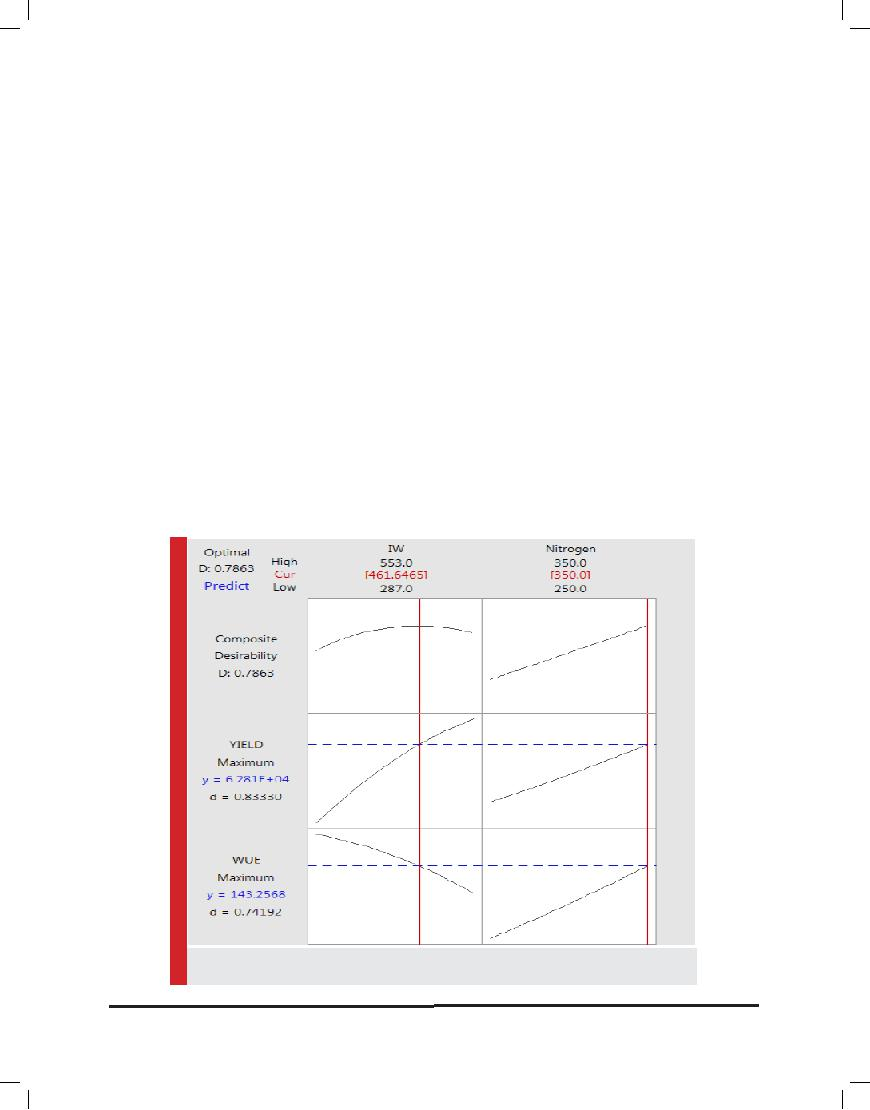values of tuber yield and water use efficiency, optimiza- tion problem was accomplished by considering the fol- lowing scenarios: In the first case, considering the lack of water resources restrictions for agriculture, the aim was to achieve maximum yield of potatoes. In the sec- ond case, the calculations were done in light of limited water resources in many parts of Iran that this is also true in the study region. The purpose of the calculations was to achieve maximum water use efficiency. Due to the overuse of nitrogen fertilizers in some potato fields, in the last scenario applying the least amount of nitro- gen fertilizer was taken into account including environ- mental considerations. In each of these situations using quadratic polynomials equations, levels of response of variables (yield and water use efficiency) were predicted based on the optimal values of the independent varia- bles (the stationary points for minimum and maximum).

FIRST SCENARIO: LACK OF WATER RESOURCES RESTRICTION

In areas where water is not a limiting factor consider- ing the lack of constraints, the aim of farming systems is to achieve the maximum performance. In this study, the maximum observational tuber yield obtained for irriga-

tion depth of 553 mm up to 69200 kg ha-1. Based on equa- tion (Brueck, 2008), it can be analytically calculate (equa- tion system 3) the depth of irrigation water and nitrogen fertilizer that can be applied to ensure maximum perfor- mance. The constraints imposed for optimizing resource, including the minimum and maximum amounts actually used in this study. Therefore using 552 mm irrigation depth maximum yield 69234 kg ha-1 was estimated. In this situation, in addition to the potato yield, if the aim is also to optimize water use efficiency, then by applying 461 mm water and 350 kg N ha-1, 62810 kg potato tubers per hectare will be harvested the return maximizing. So the maximum water use efficiency is equivalent to 143 kg ha-1 mm-1. The results showed that simultaneously maxi- mizing yield and water use efficiency with 16/5% reduc- tion in input water consumption lead to decline 9 percent in yield production. Figure 3, show optimal amounts of water and nitrogen in simultaneously maximizing the yield and water use efficiency.

SECOND SCENARIO: WATER RESOURCES RESTRICTION

Generally in arid and semi arid regions as well as the site of this research that there are water restrictions, the

FIGURE 3: Optimal values of applied Irrigation water (IW) and nitrogen for maximum yield (kg/ha) and water use efficiency (kg ha-1 mm-1)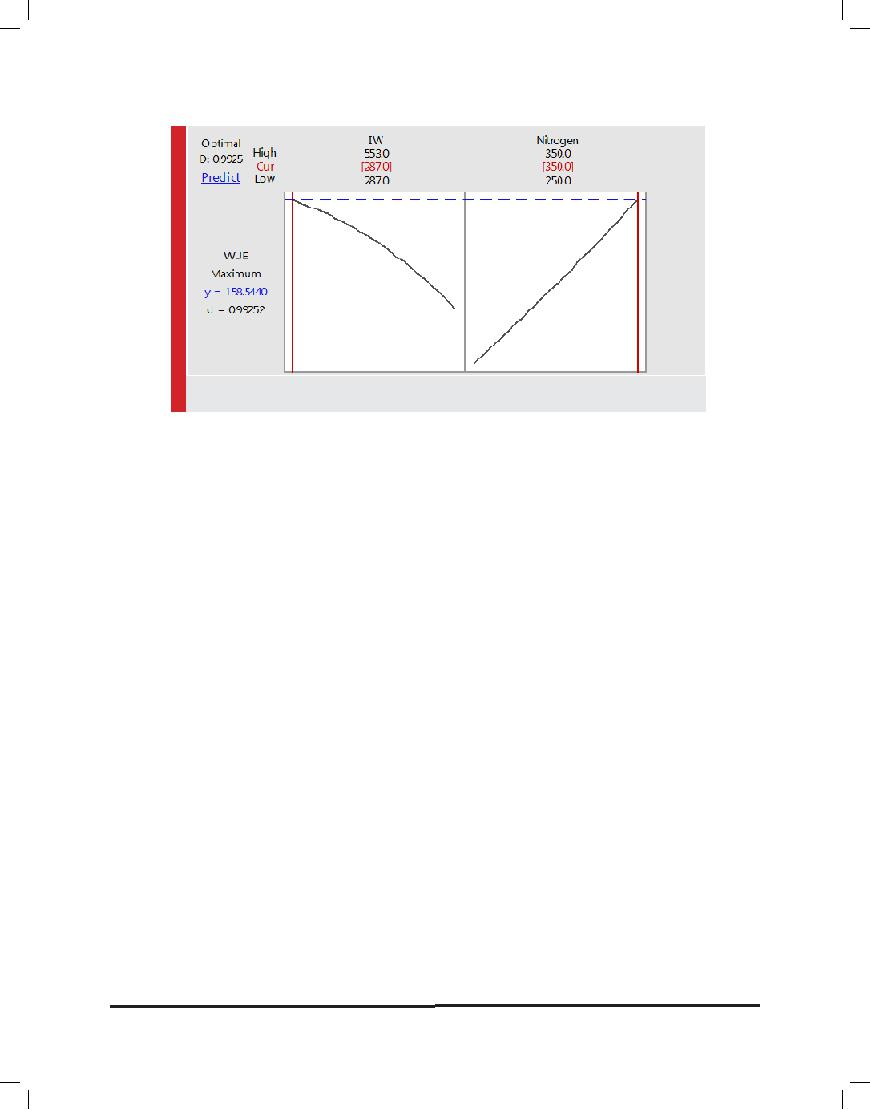FIGURE 4: Optimal values of applied Irrigation water (IW) and nitrogen for water use efficiency (kg/ha/mm)

first aim of farming systems should be to achieve maxi- mum water use efficiency. For this purpose, taking into account the minimum and maximum amounts applied for resource consumption as constraints, maximum water use efficiency for potatoes obtained by as much as 158/5 kg water ha in mm. in this case the amounts were calculated 287 mm for irrigation water and 350 kg nitrogen ha was. Namely, by applying the least amount of input water and the highest nitrogen rate, water use efficiency reached to the highest value. Minor differences between the observed maximum water use efficiency and the simulated maximum value represent- ing high accuracy of fitted model. So if the aim is to achieve the highest level of water use efficiency, then by saving 48% water, tuber yield will be diminished 38%. (Compared to full crop water requirement treatment and maximum nitrogen).

THIRD SCENARIO: APPLYING MINIMUM AMOUNT OF NITROGEN:

Environmental impact of nitrogen as well as aquifer pol- lution, which is caused by an over use of this element in farms, has led several researchers to investigate the effects of different amounts of nitrogen on yield and water use efficiency for potatoes. To assess the effect of nitrogen minimizing on yield response surfaces and water use efficiency showed that in this situation potato yield will be 50 242 kg ha-1 and water use efficiency will be about 105 kg ha-1 mm-1. In this case, that the target is the least use of nitrogen, irrigation water depth was calculated 502 mm, accordingly, with 27% reduction in tuber yield, 9% of irrigation water has been saved. In this scenario, because of environmental considerations,

for applying the least amount of nitrogen, to achieve the optimum amount of water use efficiency, (i.e. to achieve maximum water use efficiency with minimal consump- tion of nitrogen) then by applying 287 mm irrigation water and 278 kg N ha-1, water use efficiency 127 kg ha in mm-1 was estimated. Based on analysis yield losses to 51 percent of maximum Yield production so by reduc- ing 48% of irrigation water, tuber yield was up to 33931 kg per hectare. In general, results showed that reducing irrigation water and nitrogen led to deficient Tuber yield production. The results for WUE show that by reducing irrigation water depth water use efficiency increased, but by reducing the amount of nitrogen at a given depth, WUE found a descending trend. The highest water use efficiency was obtained by applying the least amount of water and the largest amount of nitrogen. Depending on the availability of water for irrigation can be adopted different strategies to achieve maximum yield or maxi- mum water use efficiency. In this regard, for reducing environmental concerns, optimal amounts of nitrogen and water can be calculated by RSM. Using fitted models even can predict Yield response surfaces and water use efficiency values corresponding to each of resources.

REFERENCES

Alva, A.K., Ren, H., and Moore, A.D. (2012). Water and nitro- gen management effects on biomass accumulation and par- titioning in two potato cultivars. American Journal of Plant Sciences, 3: 164–170.

Badr M. A., El-Tohamy W. A., and Zaghloul A. M. (2012). Yield and water use efficiency of potato grown under different irri- gation and nitrogen levels in an arid region. Agriculture Water management, 110: 9-15.Box, G.E.P., and Hunter, J.S. (1957). Multi-factor experimental designs for exploring response surfaces. Annals of Mathemati- cal Statistics, 28: 195– 241.

Box, G.E.P., and Wilson, K.B. (1951). On the experimental attainment of optimum conditions. Journal of Royal Statistical Society. Series: B. Statistical Methodology, 13: 1–45.

Brueck, H., (2008). Effects of nitrogen supply on water-use efficiency of higher plants. Journal of Plant Nutrition and Soil Science, 171: 210–219.

Darwish T., Atallah T., Hajhasan S., and Charnek A. (2003). Management of nitrogen by fertigation of potato in Lebanon. Nutrient Cycling in Agroecosystems, 67: 1-11.

Deng, X. P., Shan, L., Zhang, H., and Turner, N.C. (2006). Improving agriculture water use efficiency in arid and semi- arid areas of China. Agriculture Water Management, 80: 23–40.

Denis, B., and Boyac, H. I. (2007). Modeling and optimization

I:Usability of response surface methodology. Journal of Food Engineering, 78: 836–845.

FAO. (2012). FAOSTAT. Food and Agriculture Organization of the United Nations, Available at http://faostat3.fao.org/statis- tics.

Fathi, P., and Soltani, M. (2012). Optimization of water uses efficiency and yield in potato using marginal analysis theory. Water and Soil Resources Custody Journal, 2(2): 85-93. (in Per- sian with English abstract)

Fu, Q.P., Wang, Q. J, Shen X, L., and Fan, J. (2016). Optimizing water and nitrogen inputs for winter wheat cropping system on the Loess Plateau, China. Journal of Arid Land, 6(2): 230– 242

Hosseinpanahi, F., Mondani, F., Pouramir, F., and Nassiri Mahallati, M. (2010). Production potential of several main crops in Kurdistan province during last two decades and their future forecast. Journal of Agroecology, 2 (2): 206-210. (in Per- sian with English abstract)

Hou, L. K., Zhang, Y. J., and Zhan, J. Y. (2012). Marginal rev- enue of land and total factor productivity in Chinese agricul- ture: Evidence from spatial analysis. Journal of Geographical Sciences, 22 (1): 167–178.

Howell, T.A., Cuenca, R.H., and Solomon, K.H. (1990). Crop yield response, In: Hoffman, G.J., Howell, T.A., Solomon, K.H. (Eds), Management of Farm Irrigation Systems. ASAE. Mono- graph No. 9, 2950. Niles Road, St. Joseph, MI, pp. 99-122.

Ierna, A., Pandino, G., Lombardo, S., and Mauromicale, G. (2011). Tuber yield, water and fertilizer productivity in early potato as affected by a combination of irrigation and fertiliza- tion. Agriculture Water Management, 101: 35-41.

Kalavathy, H. M., Regupathib, I., Pillai, M. G., and Miranda, L, R. (2009). Modelling, analysis and optimization of adsorption parameters for H3PO4 activated rubber wood sawdust using response surface methodology (RSM). Colloids and Surfaces B: Biointerfaces, 70:35-45.

Kashyap, P.S., and Panda, R.K., (2003). Effect of irrigation scheduling on potato crop param-eters under water stressed conditions. Agricultural Water Management, 59: 49–66.

Koocheki, A., Nasiri, M. M., Moradi, R., and Mansouri, H. (2014). Optimizing water, nitrogen and crop density in canola cultivation using response surface methodology and central composite design. Soil Science and Plant Nutrition, 60(2): 286- 298.

Liu, W. Z., and Zhang X. C. (2015). Optimizing water and fer- tilizer input using elasticity index: A case study with Mize in the Loess plateau of china. Field Crop Research, 100: 302-310.

Liu, W. Z., Li Y.S., and Li S.X. (2012). The graphic presentation and characteristics of an optimum coupling area of water and fertilizer supply to crop. Trans. CSAE, 18(16): 1-3.

Mansouri, H., Bannayan, M., Rezvani, M. P., and Lakzian, A. (2014). Management of nitrogen fertilizer, irrigation and plant density in onion production using response surface methodol- ogy as optimization approach. African Journal of Agricultural Research, 9(7): 676-687.

Meyer, R.D., and Marcum, D.B. (1998). Potato yield, petiole nitrogen, and soil nitrogen response to water and nitrogen. Agronomy Journal, 90(3): 420–429.

Pandey, R.K., Maranville, J.W., Chetima, M.M., (2000). Deficit irrigation and nitrogen effects on maize in a Sahelian environ- ment. II. Shoot growth, nitrogen uptake and water extraction. Agricultural Water Management, 46: 15–27.

Rahil, M. H., and Qanadillo, A. (2015). Effects of different irri- gation regimes on yield and water use efficiency of cucumber crop. Agriculture Water Management, 148: 10-15.

Shen, R. K., Wang, K., Zhang, Y. F., Yang, L. H., and Mu, J. Y. (2012). Field test and study on yield, water use and N uptake under varied irrigation and fertilizer in crops. Transactions of the Chinese Society of Agricultural Engineering, 17(5): 35–38.

Waddell, J.T., Gupta, S.C., Moncrief, J.F., Rosen, C.J., and Steele, D.D. (1999). Irrigation and nitrogen management effects on potato yield tuber quality, and nitrogen uptake. Agronomy Journal, 91: 991–997.

Wang, J. P., Chen, Y.Z., Ge, X. W., and Yu, H.Q. (2014). Optimiza- tion of coagulation–flocculation process for a paper-recycling wastewater treatment using response surface methodology. Colloids and Surfaces A: Physicochemical and Engineering Aspects, 302: 204-210.

Yuan, B.Z., Nishiyama, S., and Kang, Y. (2015). Effects of dif- ferent irrigation regimes on growth and yield of drip-irrigated potato. Agricultural Water Management, 63(3): 153–167.

Zhai, B. N., and Li, S. X. (2014). Effect of water and nitrogen cooperation on winter wheat yield and quality. Plant Nutrition and Fertilizer Science, 9(1): 26–32.

Zhai, B. N., and Li, S. X. (2015). Study on the key and sensitive stage of winter wheat response to water dan nitrogen coordi- nation. Scientia Agriculutura Sinica, 38(6): 1188–

ConvertedByBCLTechnologies